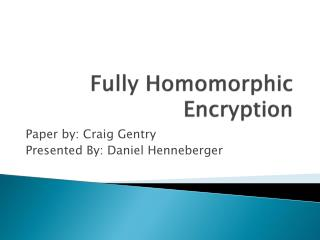DownloadDownload PresentationFully Homomorphic Encryption

# Fully Homomorphic Encryption

Download Presentation## Fully Homomorphic Encryption

- - - - - - - - - - - - - - - - - - - - - - - - - - - E N D - - - - - - - - - - - - - - - - - - - - - - - - - - -
##### Presentation Transcript

1. Fully Homomorphic Encryption Paper by: Craig Gentry Presented By: Daniel Henneberger

2. What is homomorphic encryption?

3. Homomorphic Encryption • Computations on ciphertext which predictably modifies the plaintext • Operate on messages while they are encrypted • Data can be securely processed in unsecure environments • Cloud Computing • Databases • Voting machines

4. How it works

5. How it works

6. Keygen • Encrypt • Decrypt • Evaluate

7. History • 1978 – Privacy Homomorphism • US government pumps millions in it

8. Types of Homomorphism • Additive • E(m1) + E(m2) = E(m1+m2) • Multiplicative • E(m1) * E(m2) = E(m1*m2) • Why just Add and Mul? • Can evaluate any function • Turing complete over a ring

9. Types of Homomorphism • Somewhat Homomorphic • You can do only do some functions • RSA • Fully Homomorphic • You can do all functions • Leveled Fully Homomorphic • Keysize can grow with depth of the function • Bootstrappable • Can evaluate its own decryption circuit

10. Fully Homomorphic Encryption Using Ideal Lattices Craig Gentry Stanford University and IBM Watson 2009

11. “Most unbearably complicated topic ever” –Craig Gentry

12. Importance of this topic • Before this paper, it was unknown if fully homomorphic encryption could exist • First feasible result • Holy grail of encryption • 17 results on YouTube!

13. MATH: Lattice • Ideal lattices are a form of difficult to compute mathematical problems • Similar to: • Integer Factorization • Discrete logarithm problem • Elliptic curves over finite fields (Elliptical curve) • Closest vector problem • Learning with errors • Unbreakable with quantum computing • Uses arbitrary approximations

14. Illustration - A lattice in R2borrowed from tau.ac.il Each point corresponds to a vector in the lattice “Recipe”: 1. Take two linearly independent vectors in R2. 2. Close them for addition and for multiplication by an integer scalar. etc. ... etc. ...

15. MATH: Ideal Lattice • A cyclic lattice is ‘ideal’ (ring-based) • NTRU – Asymmetric key cryptosystem that uses ring-based lattices • Low circuit complexity • Very fast • Allows additive and multiplicative homomorphism

16. More MATH • Lots of math involved with this: • Cyclotomic Polynomials • Too much for this class time

17. Advances • Evaluate(pk,C, Encrypt(pk,m1),..., Encrypt(pk,mt)) = Encrypt(pk,C(m1,..., mt)) • Steps • Create a general bootstrapping result • Initial construction using ideal lattices • Squash the decryption circuit to permit bootstrapping

18. General Bootstrapping Result

19. Initial construction using ideal lattices • Find a Public key scheme that is homomorphic for shallow circuits and uses ideal lattices • NTRUEncrypt • Ciphertext has a form of an ideal lattice + offset • Use a cyclic ring of keys • Hard to do • Large key size (GB)

20. “Squash the Decryption Circuit”

21. Bootstrap Requirements • Evaluate its own decryption circuit • Provides ability to recrypt plaintext • Must be allowed to recrypt augmented versions to provide mathematical operations

22. Improvements • Allows ‘unlimited’ additions • Recrypt algorithm • Greater multiplicative depth • log log (N) - log log (n-1) • Still bad

23. Disadvantages • Can only evaluate in logarithmic depth • Ciphertext grows • Noise increases • Addition- circuits can be corrected (recrypting) • Multiplication- noise grows quickly • Not yet practical • Client must begin the decryption process to be bootstrappable • Solution is approximate • >1 day to compute 1 message

24. Implementations • PollyCracker • Fully Homomorphic Encryption over the Integers • Fully Homomorphic Encryption over the Binary Polynomials

25. Since this paper • Many people have created new variants • Implementations • All slow • Finding shortcuts • AES-128 – Completed June 15th 2012 • Computed with 256GB of ram (still limiting factor) • 24 Xeon cores • Took 5 days per operation